Statistics

# Introduction to Descriptive Statistics: Using Mean, Median, and Standard Deviation

por Liz Roth-Johnson, Ph.D.

Imagine yourself in an introductory science course. You recently completed the first exam, and are now sitting in class waiting for your graded exam to be handed back. The course will be graded “on a curve,” so you are anxious to see how your score compares to everyone else’s. Your instructor finally arrives and shares the exam statistics for the class (see Figure 1).

The mean score is 61.

The median is 63.

The standard deviation is 12.

You receive your exam and see that you scored 72. What does this mean in relation to the rest of the class? Based on the statistics above, you can see that your score is higher than the mean and median, but how do all of these numbers relate to your final grade? In this scenario, you would end up with a “B” letter grade, even though the numerical score would equal a “C” without the curve.Figure 1: A histogram showing the distribution of exam scores for the 200 students in your class, along with the mean, median, and your score.

This scenario shows how descriptive statistics – namely the mean, median, and standard deviation – can be used to quickly summarize a dataset. By the end of this module, you will learn not only how descriptive statistics can be used to assess the results of an exam, but also how scientists use these basic statistical operations to analyze and interpret their data. Descriptive statistics can help scientists summarize everything from the results of a drug trial to the way genetic traits evolve from one generation to the next.

## What are descriptive statistics?

Descriptive statistics are used regularly by scientists to succinctly summarize the key features of a dataset or population. Three statistical operations are particularly useful for this purpose: the mean, median, and standard deviation. (For more information about why scientists use statistics in science, see our module Statistics in Science.)

### Mean vs. median

The mean and median both provide measures of the central tendency of a set of individual measurements. In other words, the mean and median roughly approximate the middle value of a dataset. As we saw above, the mean and median exam scores fell roughly in the center of the grade distribution.

Although the mean and median provide similar information about a dataset, they are calculated in different ways: The mean, also sometimes called the average or arithmetic mean, is calculated by adding up all of the individual values (the exam scores in this example) and then dividing by the total number of values (the number of students who took the exam). The median, on the other hand, is the “middle” value of a dataset. In this case, it would be calculated by arranging all of the exam scores in numerical order and then choosing the value in the middle of the dataset.

Because of the way the mean and median are calculated, the mean tends to be more sensitive to outliersvalues that are dramatically different from the majority of other values. In the example above (Figure 1), the median fell slightly closer to the middle of the grade distribution than did the mean. The 4 students who missed the exam and scored 0 (the outliers) lowered the mean by getting such different scores from the rest of the class. However, the median did not change as much because there were so few students who missed the exam compared to the total number of students in the class.

Punto de Comprensión

What is another word for the average?

### Standard deviation

The standard deviation measures how much the individual measurements in a dataset vary from the mean. In other words, it gives a measure of variation, or spread, within a dataset. Typically, the majority of values in a dataset fall within a range comprising one standard deviation below and above the mean. In the example above, the standard deviation is 12 and the majority of test scores (161 out of 200 students) scored between 49 and 73 points on the exam. If there had been more variation in the exam scores, the standard deviation would have been even larger. Conversely, if there had been less variation, the standard deviation would have been smaller. For example, let’s consider the exam scores earned by students in two different classes (Figure 2).Figure 2: Two exam score distributions with different standard deviations. Although the mean score for both classes is 50, the standard deviation, or spread, of scores is very different. The standard deviation for Class A is 5 (small spread), while the standard deviation for Class B is 15 (large spread).

In the first class (Class A – the light blue bars in the figure), all of the students studied together in a large study group and received similar scores on the final exam. In the second class (Class B – represented by dark blue bars), all of the students studied independently and received a wide range of scores on the final exam. Although the mean grade was the same for both classes (50), Class A has a much smaller standard deviation (5) than Class B (15).

Punto de Comprensión

The standard deviation is a measure of

## Normal distributionFigure 3: The distribution of exam scores shown in Figure 1 can be approximated by a normal distribution, or bell curve, which is perfectly symmetrical around the mean (dashed line).

## Early history of the normal distribution

The normal distribution is a relatively recent invention. Whereas the concept of the arithmetic mean can be traced back to Ancient Greece, the normal distribution was introduced in the early 18th century by French mathematician Abraham de Moivre. The mathematical equation for the normal distribution first appeared in de Moivre’s Doctrine of Chances, a work that broadly applied probability theory to games of chance. Despite its apparent usefulness to gamblers, de Moivre’s discovery went largely unnoticed by the scientific community for several more decades.

The normal distribution was rediscovered in the early 19th century by astronomers seeking a better way to address experimental measurement errors. Astronomers had long grappled with a daunting challenge: How do you discern the true location of a celestial body when your experimental measurements contain unavoidable instrument error and other measurement uncertainties? For example, consider the four measurements that Tycho Brahe recorded for the position of Mars shown in Table 1:Table 1:Tycho Brahe's observations of the position of Mars as presented in Saul Stahl, "The Evolution of the Normal Distribution," Mathematics Magazine 79 (April 2006), p. 99. Copyright 2006 Mathematical Association of America. All Rights Reserved. image © Mathematical Association of America

Brahe and other astronomers struggled with datasets like this, unsure how to combine multiple measurements into one “true” or representative value. The answer arrived when Carl Friedrich Gauss derived a probability distribution for experimental errors in his 1809 work Theoria motus corporum celestium. Gauss’ probability distribution agreed with previous intuitions about what an error curve should look like: It showed that small errors are more probable than large errors and that all errors are evenly distributed around the “true” value (Figure 4). Importantly, Gauss’ distribution showed that this “true” value – the most probable value in the center of the distribution – is the mean of all values in the distribution. The most probable position of Mars should therefore be the mean of Brahe’s four measurements.Figure 4: Gauss derived a probability distribution to address the inherent errors found in many experimental measurements. The “true” value (A) is the most probable value and is found in the center of the distribution. A value closer to the “true” value is more likely to be observed than a value farther away from the “true” value. For example, value B, which is close to A, is more likely to be observed than value D, which is far from A. Additionally, values are evenly distributed around the “true” value. Here, values B and C, which are both a distance “x” away from value A are equally likely to be observed.

## Further development of the normal distribution

The “Gaussian” distribution quickly gained traction, thanks in part to French mathematician Pierre-Simon Laplace. (Laplace had previously tried and failed to derive a similar error curve and was eager to demonstrate the usefulness of what Gauss had derived.)

Scientists and mathematicians soon noticed that the normal distribution could be used as more than just an error curve. In a letter to a colleague, mathematician Adolphe Quetelet noted that soldiers’ chest measurements (documented in the 1817 Edinburgh Medical and Surgical Journal) were more or less normally distributed (Figure 5). Physicist James Clerk Maxwell used the normal distribution to describe the relative velocities of gas molecules. As these and other scientists discovered, the normal distribution not only reflects experimental error, but also natural variation within a population. Today scientists use normal distributions to represent everything from genetic variation to the random spreading of molecules.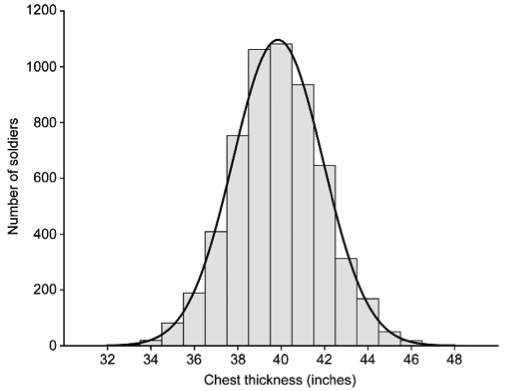Figure 5: Adolphe Quetelet noticed that the frequencies of soldiers’ chest measurements reported in the 1817 Edinburgh Medical and Surgical Journal fit a normal distribution strikingly well (though not perfectly).

## Characteristics of the normal distribution

The mathematical equation for the normal distribution may seem daunting, but the distribution is defined by only two parameters: the mean (µ) and the standard deviation (σ).

The mean is the center of the distribution. Because the normal distribution is symmetrical about the mean, the median and mean have the same value in an ideal dataset. The standard deviation provides a measure of variability, or spread, within a dataset. For a normal distribution, the standard deviation specifically defines the range encompassing 34.1% of individual measurements above the mean and 34.1% of those below the mean (Figure 6).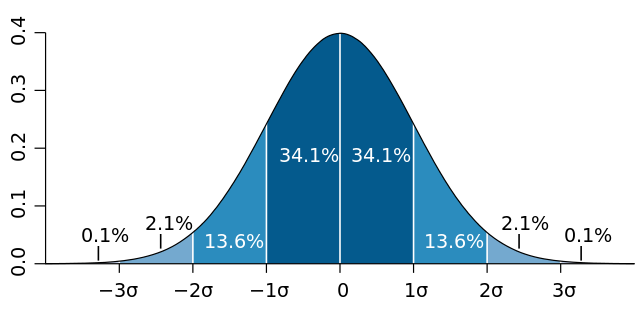Figure 6: The shape of a normal distribution is defined by the mean (µ) and the standard deviation (σ). image © Mwtoews

The concept and calculation of the standard deviation is as old as the normal distribution itself. However, the term “standard deviation” was first introduced by statistician Karl Pearson in 1893, more than a century after the normal distribution was first derived. This new terminology replaced older expressions like “root mean square error” to better reflect the value’s usefulness for summarizing the natural variation of a population in addition to the error inherent in experimental measurements. (For more on error calculation, see Statistics in Science and Uncertainty, Error, and Confidence.)

Punto de Comprensión

Normal distributions _____ commonly used in science.

## Working with statistical operations

To see how the mean, median, and standard deviation are calculated, let’s use the Scottish soldier data that originally inspired Adolphe Quetelet. The data appeared in 1817 in the Edinburgh Medical and Surgical Journal and report the “thickness round the chest” of soldiers sorted by both regiment and height (vol. 13, pp. 260 - 262). Instead of using the entire dataset, which includes measurements for 5,732 soldiers, we will consider only the 5’4’’ and 5’5’’ soldiers from the Peebles-shire Regiment (Figure 7).Figure 7: Chest width distribution for the Peebles-shire Regiment. Although the data subset of 5’4’’ and 5’5’’ soldiers (blue) does not appear to be normally distributed, it comes from a much larger dataset (gray) that can be reasonably approximated by a normal distribution. The 5'4" and 5'5" distribution of chest widths (in inches) is: 35, 35, 36, 37, 38, 38, 39, 40, 40, 40.

Note that this particular data subset does not appear to be normally distributed; however, the larger complete dataset does show a roughly normal distribution. Sometimes small data subsets may not appear to be normally distributed on their own, but belong to larger datasets that can be more reasonably approximated by a normal distribution. In such cases, it can still be useful to calculate the mean, median, and standard deviation for the smaller data subset as long as we know or have reason to assume that it comes from a larger, normally distributed dataset.

### How to calculate the mean

The arithmetic mean, or average, of a set of values is calculated by adding up all of the individual values and then dividing by the total number of values. To calculate the mean for the Peebles-shire dataset above, we start by adding up all of the values in the dataset:

35 + 35 + 36 + 37 + 38 + 38 + 39 + 40 + 40 + 40 = 378

We then divide this number by the total number of values in the dataset:

378 (sum of all values) / 10 (total number of values) = 37.8

The mean is 37.8 inches. Notice that the mean is not necessarily a value already present in the original dataset. Also notice that the mean of this dataset is smaller than the mean of the larger dataset due to the fact that we have only selected the subsample of men from the lower height group and it is reasonable to expect shorter men to be smaller overall and therefore have smaller chest widths.

### How to calculate the median

The median is the “middle” value of a dataset. To calculate the median, we must first arrange the dataset in numerical order:

35, 35, 36, 37, 38, 38, 39, 40, 40, 40

When a dataset has an odd number of values, the median is literally the median, or middle, value in the ordered dataset. When a dataset has an even number of values (as in this example), the median is the mean of the two middlemost values:

35, 35, 36, 37, 38, 38, 39, 40, 40, 40

(38 + 38) / 2 = 38

The median is 38 inches. Notice that the median is similar but not identical to the mean. Even if a data subset is itself normally distributed, the median and mean are likely to have somewhat different values.

### How to calculate the standard deviation

The standard deviation measures how much the individual values in a dataset vary from the mean. The standard deviation can be calculated in three steps:

1. Calculate the mean of the dataset. From above, we know that the mean chest width is 37.8 inches.

2. For every value in the dataset, subtract the mean and square the result.

 (35 – 37.8)2 = 7.8 (35 – 37.8)2 = 7.8 (36 – 37.8)2 = 3.2 (37 – 37.8)2 = 0.6 (38 – 37.8)2 = 0.04 (38 – 37.8)2 = 0.04 (39 – 37.8)2 = 1.4 (40 – 37.8)2 = 4.8 (40 – 37.8)2 = 4.8 (40 – 37.8)2 = 4.8

3. Calculate the mean of the values you just calculated and then take the square root.

$$( 7.8 + 7.8 + 3.2 + 0.6 + 0.04 + 0.04 + 1.4 + 4.8 + 4.8 + 4.8 ) 10 = 1.9$$

The standard deviation is 1.9 inches. The standard deviation is sometimes called the “root mean square error” because of the way it is calculated.

To concisely summarize the dataset, we could thus say that the average chest width is 37.8 ± 1.9 inches (Figure 8). This tells us both the central tendency (mean) and spread (standard deviation) of the chest measurements without having to look at the original dataset in its entirety. This is particularly useful for much larger datasets. Although we used only a portion of the Peebles-shire data above, we can just as readily calculate the mean, median, and standard deviation for the entire Peebles-shire Regiment (224 soldiers). With a little help from a computer program like Excel, we find that the average Peebles-shire chest width is 39.6 ± 2.1 inches.Figure 8. A normal curve approximation of the Peebles-shire data subset for 5’4’’ and 5’5’’ soldiers based on the mean and standard deviation values calculated above. The mean (µ), standard deviation (σ), and median (dashed black line) help to quickly and concisely summarize the data.

Punto de Comprensión

What is another word for the "middle" value of a dataset?

## Using descriptive statistics in science

As we’ve seen through the examples above, scientists typically use descriptive statistics to:

1. Concisely summarize the characteristics of a population or dataset.
2. Determine the distribution of measurement errors or experimental uncertainty.

Science is full of variability and uncertainty. Indeed, Karl Pearson, who first coined the term “standard deviation,” proposed that uncertainty is inherent in nature. (For more information about how scientists deal with uncertainty, see our module Uncertainty, Error, and Confidence). Thus, repeating an experiment or sampling a population should always result in a distribution of measurements around some central value as opposed to a single value that is obtained each and every time. In many (though not all) cases, such repeated measurements are normally distributed.

Descriptive statistics provide scientists with a tool for representing the inherent uncertainty and variation in nature. Whether a physicist is taking extremely precise measurements prone to experimental error or a pharmacologist is testing the variable effects of a new medication, descriptive statistics help scientists analyze and concisely represent their data.

## Sample problem 1

An atmospheric chemist wants to know how much an interstate freeway contributes to local air pollution. Specifically, she wants to measure the amount of fine particulate matter (small particles less than 2.5 micrometers in diameter) in the air because this type of pollution has been linked to serious health problems. The chemist measures the fine particulate matter in the air (measured in micrograms per cubic meter of air) both next to the freeway and 10 miles away from the freeway. Because she expects some variability in her measurements, she samples the air several times every day. Here is a representative dataset from one day of sampling:

Fine particulate matter in the air (µg/m3)
Next to the freeway 10 miles away from the freeway
29.3 11.8
18.3 12.5
17.7 13.1
17.9 9.6
18.9 14.6
20.9 10.4
18.6 9.8

Help the atmospheric chemist analyze her findings by calculating the mean (µ) and standard deviation (σ) for each dataset. What can she conclude about freeway contribution to air pollution? (Problem modeled loosely after Phuleria et al., 2007)

### Solution 1

$$μ = ( 19.3 + 18.3 + 17.7 + 17.9 + 18.9 + 20.9 + 18.6 ) 7 = 18.8$$

$$σ = ( 19.3 − 18.8 ) 2 + ( 18.3 − 18.8 ) 2 + ( 17.7 − 18.8 ) 2 + ( 17.9 − 18.8 ) 2 + ( 18.9 − 18.8 ) 2 + ( 20.9 − 18.8 ) 2 + ( 18.6 − 18.8 ) 2 7 = 1.0$$

Now we can do the same procedure for the dataset collected 10 miles away from the freeway:

$$μ = ( 11.8 + 12.5 + 13.1 + 9.6 + 14.6 + 10.4 + 9.8 ) 7 = 11.7$$

$$σ = ( 11.8 − 11.7 ) 2 + ( 12.5 − 11.7 ) 2 + ( 13.1 − 11.7 ) 2 + ( 9.6 − 11.7 ) 2 + ( 14.6 − 11.7 ) 2 + ( 10.4 − 11.7 ) 2 + ( 9.8 − 11.7 ) 2 7 = 1.7$$

There is 18.8 ± 1.0 µg/m3 fine particulate matter next to the freeway versus 11.7 ± 1.7 µg/m3 10 miles away from the freeway. The atmospheric chemist can conclude that there is much more air pollution next to the freeway than far away.

## Sample problem 2

A climatologist at the National Climate Data Center is comparing the climates of different cities across the country. In particular, he would like to compare the daily maximum temperatures for 2014 of a coastal city (San Diego, CA) and an inland city (Madison, WI). He finds the daily maximum temperature measurements recorded for each city throughout the year 2014 and loads them into an Excel spreadsheet. Using the functions built into Excel, help the climatologist summarize and compare the two datasets by calculating the median, mean, and standard deviation.

### Solution 2

Download and open the Excel file containing the daily maximum temperatures for Madison, WI (cells B2 through B366) and San Diego, CA (cells C2 through C366). (Datasets were retrieved from the National Climate Data Center http://www.ncdc.noaa.gov/)

To calculate the median of the Madison dataset, click on an empty cell, type “=MEDIAN(B2:B366)” and hit the enter key. This is an example of an Excel “function,” and it will calculate the median of all of the values contained within cells B2 through B366 of the spreadsheet.

The same procedure can be used to calculate the mean of the Madison dataset by typing a different function “=AVERAGE(B2:B366)” in an empty cell and pressing enter.

To calculate the standard deviation, type the function “=STDEV.P(B2:B366)” and press enter. (Older versions of Excel will use the function STDEVP instead.)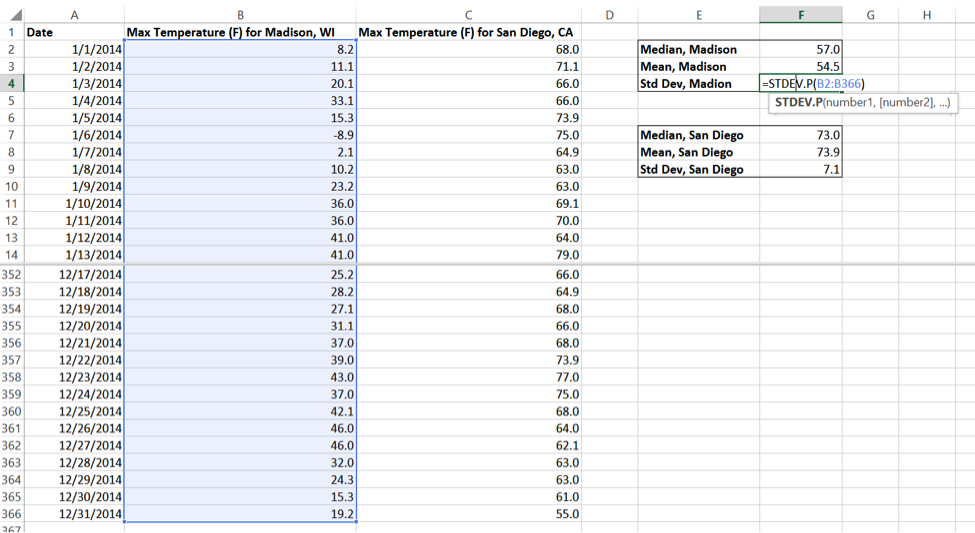Excel page 4: Calculating the median, mean, and standard deviation of the San Diego dataset

The same procedure can be used to calculate the median, mean, and standard deviation of the San Diego dataset in cells C2 through C366.

On average, Madison is much colder than San Diego: In 2014, Madison had a mean daily maximum temperature of 54.5°F and a median daily maximum temperature of 57°F. In contrast, San Diego had a mean daily maximum temperature of 73.9°F and a median daily maximum temperature of 73°F. Madison also had much more temperature variability throughout the year compared to San Diego. Madison’s daily maximum temperature standard deviation was 23.8°F, while San Diego’s was only 7.1°F. This makes sense, considering that Madison experiences much more seasonal variation than San Diego, which is typically warm and sunny all year round.

## Non-normal distributions

Not all datasets are normally distributed. Because the world population is steadily increasing, the global age appears as a skewed distribution with more young people than old people (Figure 9). Unlike the normal distribution, this distribution is not symmetrical about the mean. Because it is impossible to have an age below zero, the left side of the distribution stops abruptly while the right side of the distribution trails off gradually as the age range increases.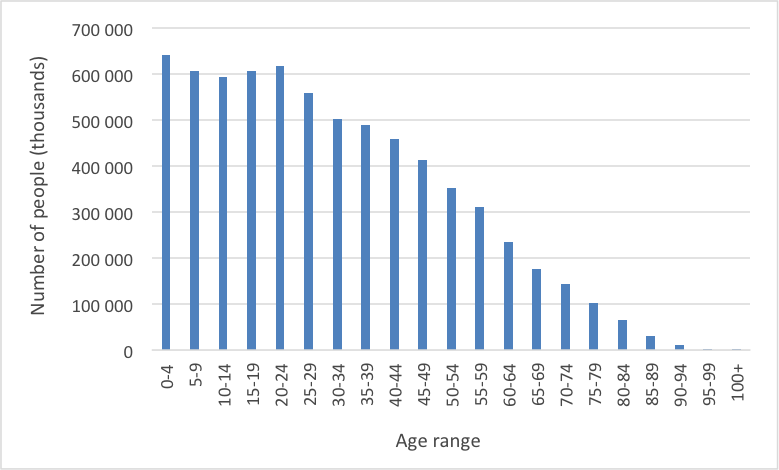Figure 9: Global age distribution for the year 2010. Data from UN Population Division, http://www.un.org/en/development/desa/population/

Distributions with multiple, distinct peaks can also emerge from mixed populations. Evolutionary biologists studying the beak sizes of Darwin’s finches in the Galapagos Islands have observed a bimodal distribution of finches (Figure 10).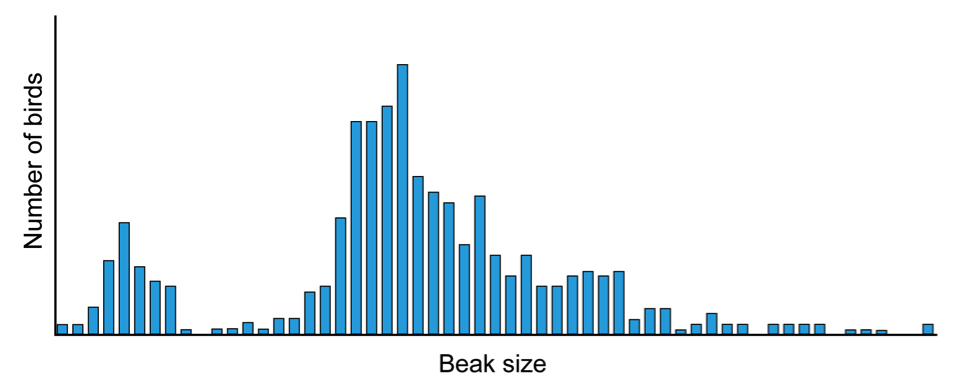Figure 10: Distribution of relative beak sizes among three species of finches in the Galapagos. Notice how there are two clear populations of finches: one with smaller beaks and one with larger beaks. (Based on Hendry et al., 2009.)

In fact, the term “normal distribution” is quite misleading, because it implies that all other distributions are somehow abnormal. Many different types of distributions are used in science and help scientists summarize and interpret their data.

### Resumen

Scientists look to uncover trends and relationships in data. This is where descriptive statistics is an important tool, allowing scientists to quickly summarize the key characteristics of a population or dataset. The module explains median, mean, and standard deviation and explores the concepts of normal and non-normal distribution. Sample problems show readers how to perform basic statistical operations.

### Conceptos Clave

• Basic statistical operations such as mean, median, and standard deviation help scientists quickly summarize the major characteristics of a dataset.

• A normal distribution is a type of probability distribution in which the probability of observing any specific value is evenly distributed about the mean of the dataset. In many scientific applications, the statistical error in experimental measurements and the natural variation within a population are approximated as normal distributions.

• Standard deviation provides a measurement of the “spread” of a dataset, or how much individual values in a dataset vary from the mean. This “spread” of data helps scientists summarize how much variation there is in a dataset or population.

• HS-C1.4
• ##### Referencias
• Critical review of the state of medicine during the last ten years. (1817). In Edinburgh Medical and Surgical Journal. Edinburgh: A. and C. Black: 1-68.
• de Moivre, A. (1718). The doctrine of chances: A method of calculating the probability of events in play, 2nd ed. London: W. Pearson. Reprinted (1967) New York: Chelsea Publishing Co.
• Gauss, K. F. (1809). Theoria motus corporum celestium. Hamburg: Perthes. English translation (1963), Theory of the motion of the heavenly bodies moving about the sun in conic sections. New York: Dover Publications, Inc.
• Hendry, A. P., Huber, S. K., De Leon, L. F., Herrel, A., & Podos, J. (2009). Disruptive selection in a bimodal population of Darwin’s finches. Proceedings of Biological Science, 276(1657), 753-759.
• Jaynes, E. T. (1995). Probability theory: The logic of science. St. Louis, MO: Washington University.
• Maxwell, J. C. (1860). Illustrations of the dynamical theory of gases. Philosophical Magazine Series 4, 19(124), 19-32. Reprinted in The Scientific Papers of James Clerk Maxwell (1952). New York: Dover.
• Phuleria, H. C., Sheesley, R. J., Schauer, J. J., Fine, P. M., & Sioutas, C. (2007). Roadside measurements of size-segregated particulate organic compounds near gasoline and diesel-dominated freeways in Los Angeles, CA. Atmospheric Environment, 41(22), 4653-4671.
• Quetelet, A. (1846). Lettres à S.A.R. le duc régnant de Saxe-Coburg et Gotha, sur la théorie des probabilités, appliquée aux sciences morales et politiques. Brussels: Hayez.
• Stahl, S. (2006). The evolution of the normal distribution. Mathematics Magazine, 79(2), 96–113.

Liz Roth-Johnson, Ph.D. “Introduction to Descriptive Statistics” Visionlearning Vol. MAT-3 (4), 2015.

Top